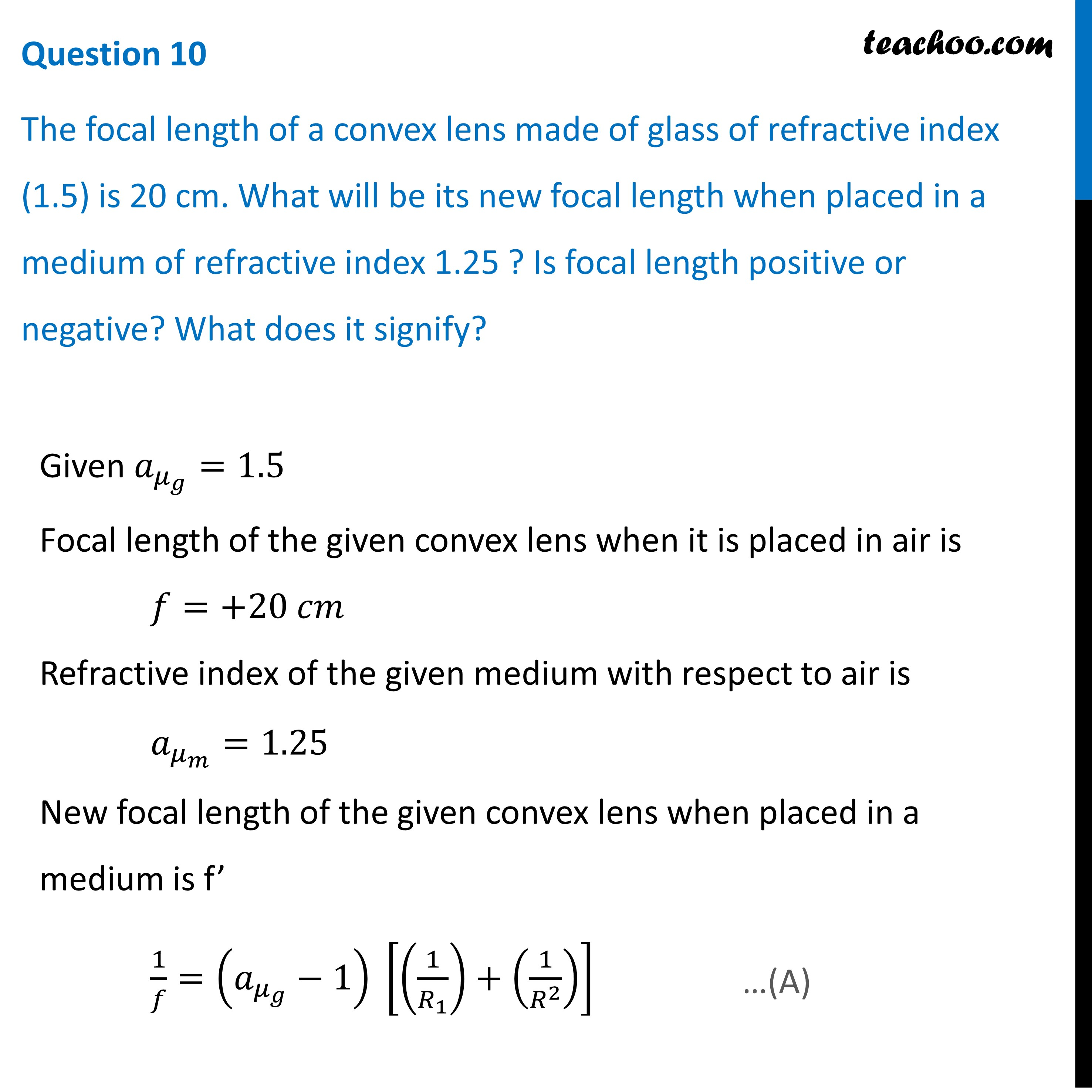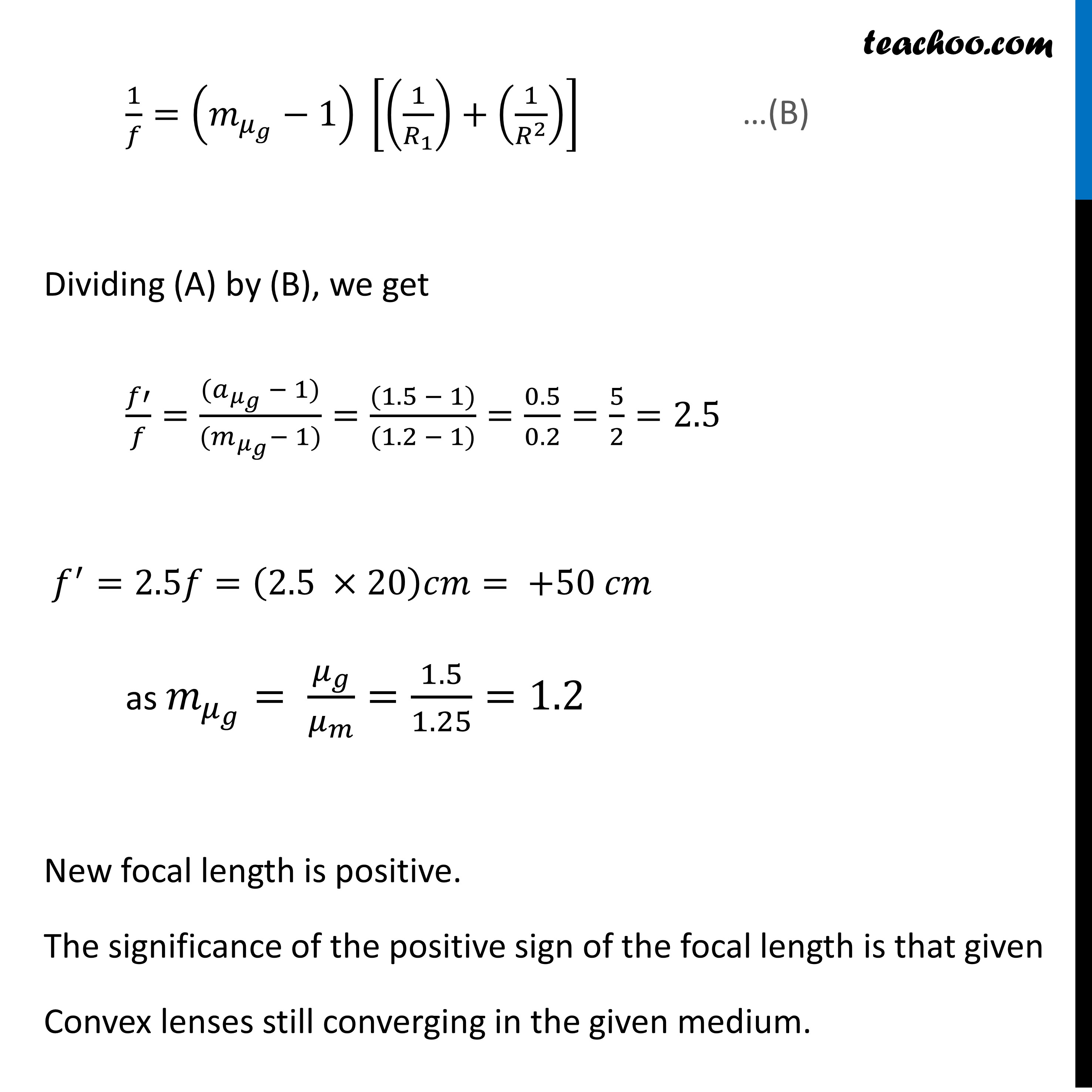CBSE Class 12 Sample Paper for 2022 Boards [Term 2] - Physics

Physics Class 12
Solutions to CBSE Sample Paper - Physics Class 12

## The focal length of a convex lens made of glass of refractive index (1.5) is 20 cm. What will be its new focal length when placed in a medium of refractive index 1.25 ? Is focal length positive or negative? What does it signify?Learn in your speed, with individual attention - Teachoo Maths 1-on-1 Class

### Transcript

Question 10 The focal length of a convex lens made of glass of refractive index (1.5) is 20 cm. What will be its new focal length when placed in a medium of refractive index 1.25 ? Is focal length positive or negative? What does it signify? Given 𝑎_(𝜇_𝑔 )=1.5 Focal length of the given convex lens when it is placed in air is 𝑓=+20 𝑐𝑚 Refractive index of the given medium with respect to air is 𝑎_(𝜇_𝑚 )=1.25 New focal length of the given convex lens when placed in a medium is f’ 1/𝑓=(𝑎_(𝜇_𝑔 )−1) [(1/𝑅_1 )+(1/𝑅^2 )] Given 𝑎_(𝜇_𝑔 )=1.5 Focal length of the given convex lens when it is placed in air is 𝑓=+20 𝑐𝑚 Refractive index of the given medium with respect to air is 𝑎_(𝜇_𝑚 )=1.25 New focal length of the given convex lens when placed in a medium is f’ 1/𝑓=(𝑎_(𝜇_𝑔 )−1) [(1/𝑅_1 )+(1/𝑅^2 )] 1/𝑓=(𝑚_(𝜇_𝑔 )−1) [(1/𝑅_1 )+(1/𝑅^2 )] Dividing (A) by (B), we get 𝑓′/𝑓=((𝑎_(𝜇_𝑔 ) − 1))/((𝑚_(𝜇_𝑔 )− 1))=((1.5 − 1))/((1.2 − 1))=0.5/0.2=5/2=2.5 𝑓^′=2.5𝑓=(2.5 ×20)𝑐𝑚= +50 𝑐𝑚 as 𝑚_(𝜇_𝑔 )= 𝜇_𝑔/𝜇_𝑚 =1.5/1.25=1.2 New focal length is positive. The significance of the positive sign of the focal length is that given Convex lenses still converging in the given medium.